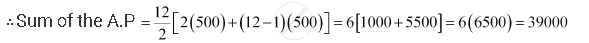Share

# A Farmer Buys a Used Tractor for Rs 12000. He Pays Rs 6000 Cash and Agrees to Pay the Balance in Annual Installments of Rs 500 Plus 12% Interest on the Unpaid Amount. How Much Will Be the Tractor Cost Him? - Mathematics

#### Question

A farmer buys a used tractor for Rs 12000. He pays Rs 6000 cash and agrees to pay the balance in annual installments of Rs 500 plus 12% interest on the unpaid amount. How much will be the tractor cost him?

#### Solution

It is given that the farmer pays Rs 6000 in cash.

Therefore, unpaid amount = Rs 12000 – Rs 6000 = Rs 6000

According to the given condition, the interest paid annually is

12% of 6000, 12% of 5500, 12% of 5000, …, 12% of 500

Thus, total interest to be paid = 12% of 6000 + 12% of 5500 + 12% of 5000 + … + 12% of 500

= 12% of (6000 + 5500 + 5000 + … + 500)

= 12% of (500 + 1000 + 1500 + … + 6000)

Now, the series 500, 1000, 1500 … 6000 is an A.P. with both the first term and common difference equal to 500.

Let the number of terms of the A.P. be n.

∴ 6000 = 500 + (n – 1) 500

⇒ 1 + (n – 1) = 12

⇒ n = 12Thus, total interest to be paid = 12% of (500 + 1000 + 1500 + … + 6000)

= 12% of 39000 = Rs 4680

Thus, cost of tractor = (Rs 12000 + Rs 4680) = Rs 16680

Is there an error in this question or solution?

#### APPEARS IN

NCERT Solution for Mathematics Textbook for Class 11 (2018 (Latest))
Chapter 9: Sequences and Series
Q: 27 | Page no. 200

#### Video TutorialsVIEW ALL 

Solution A Farmer Buys a Used Tractor for Rs 12000. He Pays Rs 6000 Cash and Agrees to Pay the Balance in Annual Installments of Rs 500 Plus 12% Interest on the Unpaid Amount. How Much Will Be the Tractor Cost Him? Concept: Arithmetic Progression (A.P.).
S